# Adding and Subtracting Positive and Negative Numbers

Author: Sophia Tutorial
##### Description:

Evaluate a sum or difference with positive and negative numbers.

(more)

Sophia’s self-paced online courses are a great way to save time and money as you earn credits eligible for transfer to many different colleges and universities.*

No credit card required

37 Sophia partners guarantee credit transfer.

299 Institutions have accepted or given pre-approval for credit transfer.

* The American Council on Education's College Credit Recommendation Service (ACE Credit®) has evaluated and recommended college credit for 33 of Sophia’s online courses. Many different colleges and universities consider ACE CREDIT recommendations in determining the applicability to their course and degree programs.

Tutorial
what's covered
1. Adding Positive and Negative Numbers
2. Subtracting Positive and Negative Numbers

# 1. Adding Positive and Negative Numbers

The ability to work comfortably with negative numbers is essential to success in algebra. For this reason, we will do a quick review of adding and subtracting positive and negative integers. Integers are all the positive whole numbers, zero, and their opposites (negative numbers).

When adding integers, we have two cases to consider. The first case demonstrates a situation with matching signs: both integers are either positive, or both integers are negative. If the signs match, we will add the numbers together, and keep the sign. This is illustrated in the following examples: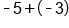Same sign, add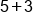, keep the negative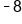Our Solution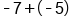Same sign, add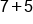, keep the negative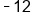Our Solution

The second case demonstrates a situation with signs that don't match (one integers is positive and one integer is negative). We will subtract the numbers (as if they were all positive), and then use the sign from the larger number. This means if the larger number is positive, the answer is positive; and if the larger numbers is negative, the answer is negative. This is shown in the following examples: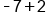Different signs, subtract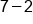, use sign from bigger number, negative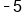Our Solution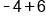Different signs, subtract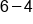, use sign from bigger number, positive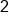Our Solution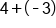Different signs, subtract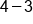, use sign from bigger number, positive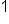Our Solution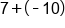Different signs, subtract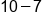, use sign from bigger number, negative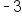Our Solution

big idea
When adding two numbers with matching signs, add the two numbers (as if they are positive) and keep the sign. When adding two numbers with opposite signs, subtract the smaller number from the larger number (as if they are positive), and keep the sign of the larger number.

# 2. Subtracting Positive and Negative numbers

For subtraction of negative numbers, we will change the problem to an addition problem, which can then be solved using the above methods. The way we change subtraction to addition is to add the opposite of the number after the subtraction sign. Often this method is referred to "adding the opposite." This is illustrated in the following examples: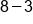Add the opposite of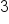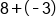Different signs, subtract, use sign from bigger number, positive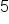Our Solution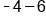Add the opposite of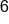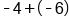Same sign, add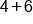, keep the negative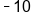Our Solution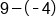Add the opposite of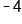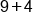Same sign, add, keep the positive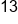Our Solution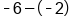Add the opposite of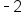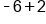Different sign, subtract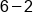, use sign from bigger number, negativeOur Solution

summary
When adding and subtracting positive and negative numbers, it is important to pay attention to the sign of the numbers. When subtracting integers, it is often helpful to rewrite as addition. To rewrite a subtraction problem as addition, change the sign of the number after the subtraction sign, and change the operation from subtraction to addition. Then, we can follow strategies for adding positive and negative numbers.

Source: ADAPTED FROM "BEGINNING AND INTERMEDIATE ALGEBRA" BY TYLER WALLACE, AN OPEN SOURCE TEXTBOOK AVAILABLE AT: HTTP://WALLACE.CCFACULTY.ORG/BOOK/BOOK.HTML

Rating